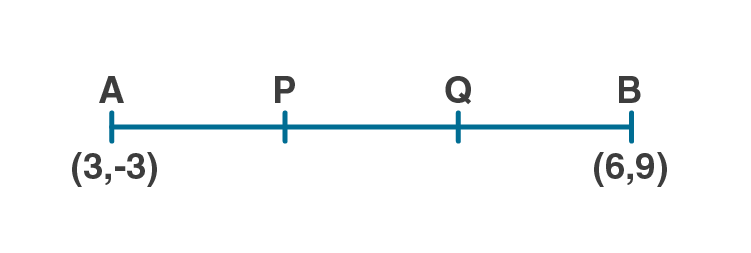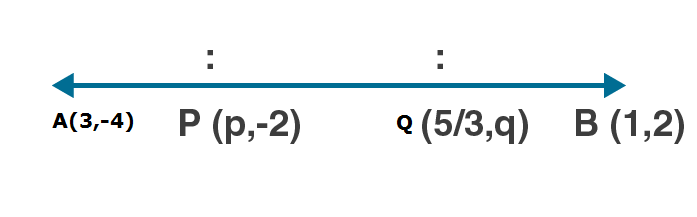Newbie

# (i) Find the co-ordinates of the points of trisection of the line segment joining the point (3, – 3) and (6, 9). (ii) The line segment joining the points (3, – 4) and (1, 2) is trisected at the points P and Q. If the coordinates of P and Q are (p, – 2) and (5/3, q) respectively, find the values of p and q.

• 0

M.L Aggarwal book Important Question of class 10 chapter Based on Section Formula for ICSE BOARD.
In this question co-ordinates are given you have to solve this question as asked in question.
This is the Question Number 04, Exercise 11 of M.L Aggarwal

Share

1.Let P and Q be the points of trisection of AB

i.e., AP = PQ = QB

Given A(3,-3) and B(6,9)

x1 = 3, y1 = -3, x2 = 6, y2 = 9

P(x, y) divides AB internally in the ratio 1 : 2.

m:n = 1:2

By applying the section formula, the coordinates of P are as follows.

By Section formula x = (mx2+nx1)/(m+n)

x = (1×6+2×3)/(1+2)

x = (6+6)/3

x = 12/3

x = 4

By Section formula y = (my2+ny1)/(m+n)

y = (1×9+2×-3)/(2+1)

y = (9-6)/3

y = 3/3

y = 1

Hence the co-ordinate of point P are (4,1).

Now, Q also divides AB internally in the ratio 2 : 1.

m:n = 2:1

By applying the section formula, the coordinates of P are as follows.

By Section formula x = (mx2+nx1)/(m+n)

x = (2×6+1×3)/(1+2)

x = (12+3)/3

x = 15/3

x = 5

By Section formula y = (my2+ny1)/(m+n)

y = (2×9+1×-3)/(2+1)

y = (18-3)/3

y = 15/3

y = 5

Hence the co-ordinate of point Q are (5,5).

(ii) Let P(p,-2) and Q(5/3, q) be the points of trisection of ABi.e., AP = PQ = QB

Given A(3,-4) and B(1,2)

x1 = 3, y1 = -4, x2 = 1, y2 = 2

P(p, -2) divides AB internally in the ratio 1 : 2.

By Section formula x = (mx2+nx1)/(m+n)

p = (1×1+2×3)/(1+2)

p = (1+6)/3

p = 7/3

Now, Q also divides AB internally in the ratio 2 : 1.

m:n = 2:1

Q(5/3, q) divides AB internally in the ratio 2 : 1.

By Section formula y = (my2+ny1)/(m+n)

q = (2×2+1×-4)/(2+1)

q = (4-4)/3

q = 0/3

q = 0

Hence the value of p and q are 7/3 and 0 respectively.

• 0